# Test: Network Equation (Mesh & Node) - 1

## 10 Questions MCQ Test Network Theory (Electric Circuits) | Test: Network Equation (Mesh & Node) - 1

Description
Attempt Test: Network Equation (Mesh & Node) - 1 | 10 questions in 30 minutes | Mock test for Electrical Engineering (EE) preparation | Free important questions MCQ to study Network Theory (Electric Circuits) for Electrical Engineering (EE) Exam | Download free PDF with solutions
QUESTION: 1

### v1 = ?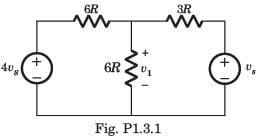Solution:

Applying the nodal analysis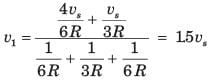QUESTION: 2

### va = ?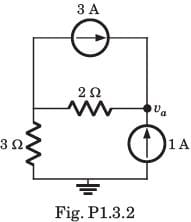Solution:

va = 2(3 + 1) + 3 (1) = 11 V

QUESTION: 3

### v1 = ?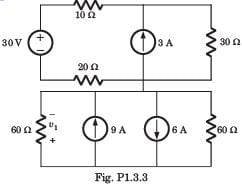Solution: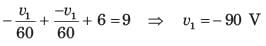QUESTION: 4

va = ?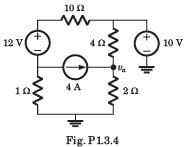Solution: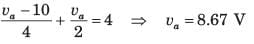QUESTION: 5

v2 = ?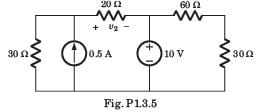Solution: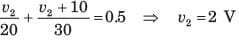QUESTION: 6

ib = ?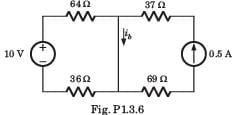Solution:

Explanation : Current due to voltage source is:

I' = 10/(64+36)

= 0.1A.

Current due to current source is:

I = 0.5A.

Current ib = I' + I

= 0.5+ 0.1

= 0.6A

QUESTION: 7

Find the power supplied by the dependent voltage source in the circuit given below.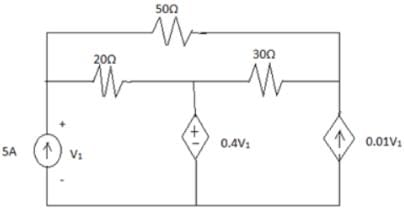Solution:

3loops = 3KVL equations. Solving them gives currents flowing in the circuit. I1 = 5A, I2 = -1.47A, I3 = 0.56A. Power supplied by dependent voltage source = 0.4V1 (I1 - I2).

QUESTION: 8

i1 = ?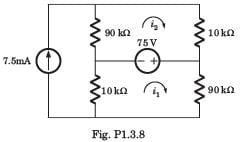Solution: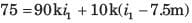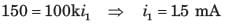QUESTION: 9

i1 = ?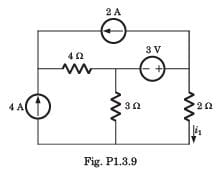Solution: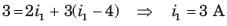QUESTION: 10

i1 = ?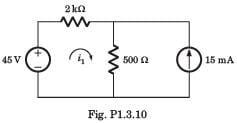Solution: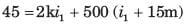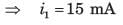Use Code STAYHOME200 and get INR 200 additional OFF Use Coupon Code